IMO Shortlist 2013 problem C5

Kvaliteta:
Avg: 0,0
Težina:
Avg: 8,0
Let$r$ be a positive integer, and let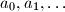$a_0, a_1, \ldots$ be an infinite sequence of real numbers. Assume that for all nonnegative integers$m$ and$s$ there exists a positive integer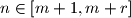$n \in [m + 1, m + r]$ such that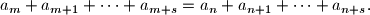Prove that the sequence is periodic, i.e. there exists some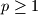$p \geq 1$ such that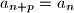$a_{n+p} = a_n$ for all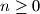$n \geq 0$.
Izvor: India